## Standard Notation for Defining Sets

### Learning Outcomes

• Write sets using set-builder, inequality, and interval notation
• Describe sets on the real number line using set builder, interval, and inequality notation

There are several ways to define sets of numbers or mathematical objects. The reason we are introducing this here is because we often need to define the sets of numbers that make up the inputs and outputs of a function.

How we write sets that make up the domain and range of functions often depends on how the relation or function are defined or presented to us.  For example, we can use lists to describe the domain of functions that are given as sets of ordered pairs. If we are given an equation or graph, we might use inequalities or intervals to describe domain and range.

In this section, we will introduce the standard notation used to define sets, and give you a chance to practice writing sets in three ways, inequality notation, set-builder notation, and interval notation.

Consider the set $\left\{x|10\le x<30\right\}$, which describes the behavior of $x$ in set-builder notation. The braces $\{\}$ are read as “the set of,” and the vertical bar $|$ is read as “such that,” so we would read $\left\{x|10\le x<30\right\}$ as “the set of x-values such that 10 is less than or equal to $x$, and $x$ is less than 30.”

The table below compares inequality notation, set-builder notation, and interval notation.

Inequality Notation Set-builder Notation Interval Notation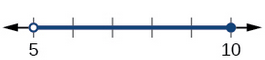$5<h\le10$ $\{h | 5 < h \le 10\}$ $(5,10]$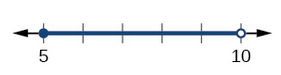$5\le h<10$ $\{h | 5 \le h < 10\}$ $[5,10)$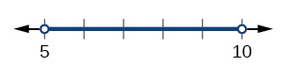$5<h<10$ $\{h | 5 < h < 10\}$ $(5,10)$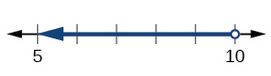$h<10$ $\{h | h < 10\}$ $(-\infty,10)$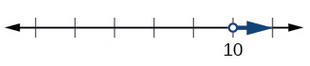$h>10$ $\{h | h > 10\}$ $(10,\infty)$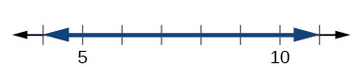All real numbers $\mathbf{R}$ $(−\infty,\infty)$

To combine two intervals using inequality notation or set-builder notation, we use the word “or.” As we saw in earlier examples, we use the union symbol, $\cup$, to combine two unconnected intervals. For example, the union of the sets $\left\{2,3,5\right\}$ and $\left\{4,6\right\}$ is the set $\left\{2,3,4,5,6\right\}$. It is the set of all elements that belong to one or the other (or both) of the original two sets. For sets with a finite number of elements like these, the elements do not have to be listed in ascending order of numerical value. If the original two sets have some elements in common, those elements should be listed only once in the union set. For sets of real numbers on intervals, another example of a union is

$\left\{x|\text{ }|x|\ge 3\right\}=\left(-\infty ,-3\right]\cup \left[3,\infty \right)$

This video describes how to use interval notation to describe a set.

This video describes how to use Set-Builder notation to describe a set.

### A General Note: Set-Builder Notation and Interval Notation

Set-builder notation is a method of specifying a set of elements that satisfy a certain condition. It takes the form $\left\{x|\text{statement about }x\right\}$ which is read as, “the set of all $x$ such that the statement about $x$ is true.” For example,

$\left\{x|4<x\le 12\right\}$

Interval notation is a way of describing sets that include all real numbers between a lower limit that may or may not be included and an upper limit that may or may not be included. The endpoint values are listed between brackets or parentheses. A square bracket indicates inclusion in the set, and a parenthesis indicates exclusion from the set. For example,

$\left(4,12\right]$

### How To: Given a line graph, describe the set of values using interval notation.

1. Identify the intervals to be included in the set by determining where the heavy line overlays the real line.
2. At the left end of each interval, use [ with each end value to be included in the set (solid dot) or ( for each excluded end value (open dot).
3. At the right end of each interval, use ] with each end value to be included in the set (filled dot) or ) for each excluded end value (open dot).
4. Use the union symbol $\cup$ to combine all intervals into one set.

### Example: Describing Sets on the Real-Number Line

Describe the intervals of values shown below using inequality notation, set-builder notation, and interval notation.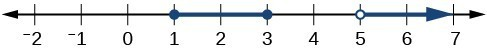### Try It

Given the graph below, specify the graphed set in

1. words
2. set-builder notation
3. interval notation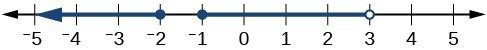The table below gives a summary of interval notation.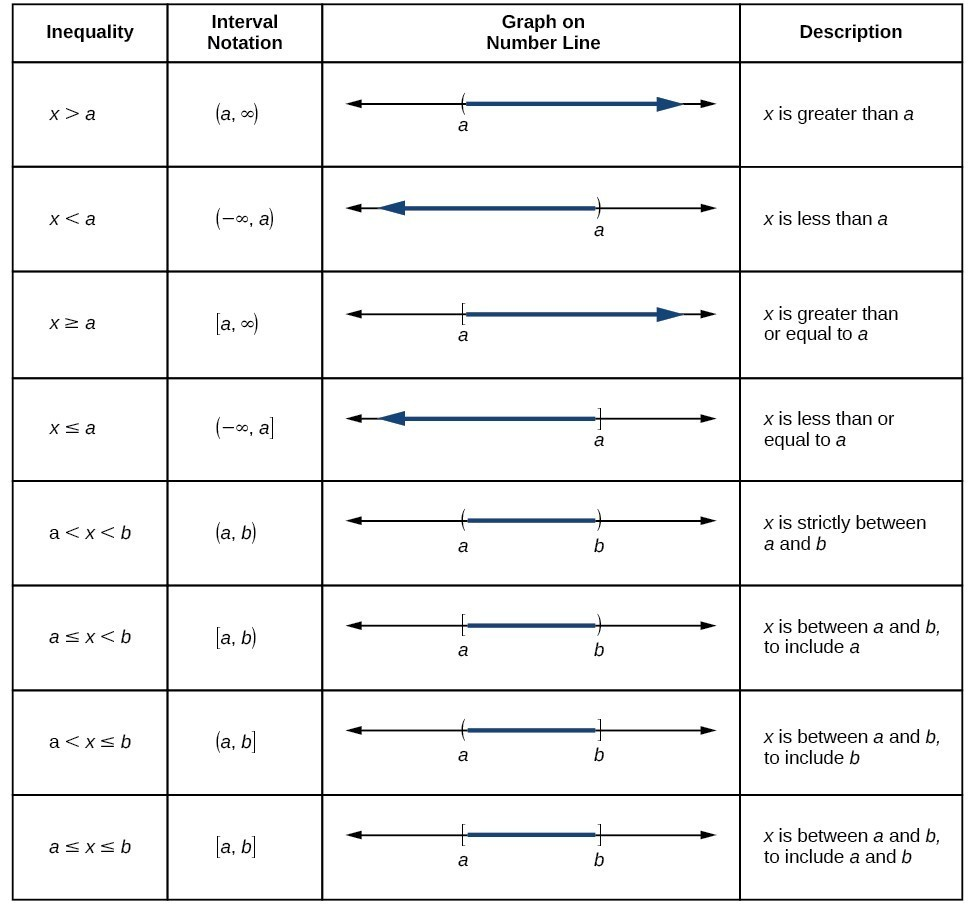## Contribute!

Did you have an idea for improving this content? We’d love your input.